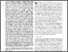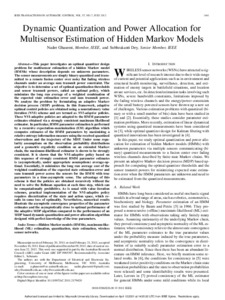# Dynamic Quantization and Power Allocation for Multisensor Estimation of Hidden Markov Models

Ghasemi, Nader and Dey, Subhrakanti (2012) Dynamic Quantization and Power Allocation for Multisensor Estimation of Hidden Markov Models. IEEE Transactions on Automatic Control, 57 (7). pp. 1641-1656. ISSN 0018-9286Previewmore...Add this article to your Mendeley library

## Abstract

This paper investigates an optimal quantizer design problem for multisensor estimation of a hidden Markov model (HMMs) whose description depends on unknown parameters. The sensor measurements are simply binary quantized and transmitted to a remote fusion center over noisy flat fading wireless channels under an average sum transmit power constraint. The objective is to determine a set of optimal quantization thresholds and sensor transmit powers, called an optimal policy, which minimizes the long run average of a weighted combination of the expected state estimation error and sum transmit power. We analyze the problem by formulating an adaptive Markov decision process (MDP) problem. In this framework, adaptive optimal control policies are obtained using a nonstationary value iteration (NVI) scheme and are termed as NVI-adaptive policies. These NVI-adaptive policies are adapted to the HMM parameter estimates obtained via a strongly consistent maximum likelihood estimator. In particular, HMM parameter estimation is performed by a recursive expectation-maximization (EM) algorithm which computes estimates of the HMM parameters by maximizing a relative entropy information measure using the received quantized observations and the trajectory of the MDP. Under some regularity assumptions on the observation probability distributions and a geometric ergodicity condition on an extended Markov chain, the maximum-likelihood estimator is shown to be strongly consistent. It is shown that the NVI-adaptive policy based on this sequence of strongly consistent HMM parameter estimates is (asymptotically, under appropriate assumptions) average-optimal. Essentially, it minimizes the long run average cost of the weighted combination of the expected state estimation error and sum transmit power across the sensors for the HMM with true parameters in a time-asymptotic sense. The advantage of this scheme is that the policies are obtained recursively without the need to solve the Bellman equation at each time step, which can be computationally prohibitive. As is usual with value iteration schemes, practical implementation of the NVI-adaptive policy requires discretization of the state and action space, which results in some loss of optimality. Nevertheless, numerical results illustrate the asymptotic convergence properties of the parameter estimates and the asymptotically close to optimal performance of the adaptive MDP algorithm compared to the performance of an MDP based dynamic quantization and power allocation algorithm designed with perfect knowledge of the true parameters.

Item Type: Article Cite as: N. Ghasemi and S. Dey, "Dynamic Quantization and Power Allocation for Multisensor Estimation of Hidden Markov Models," in IEEE Transactions on Automatic Control, vol. 57, no. 7, pp. 1641-1656, July 2012, doi: 10.1109/TAC.2011.2179420. Hidden Markov models (HMMs); maximum-likelihood (ML) estimation; quantization; state estimation; wireless sensor networks; Faculty of Science and Engineering > Electronic EngineeringFaculty of Science and Engineering > Research Institutes > Hamilton Institute 14324 https://doi.org/10.1109/TAC.2011.2179420 Subhrakanti Dey 12 Apr 2021 14:27 IEEE Transactions on Automatic Control IEEE YesItem control page